# Catalysis - Chemical Kinetics Chemistry Notes | EduRev

## Chemistry : Catalysis - Chemical Kinetics Chemistry Notes | EduRev

The document Catalysis - Chemical Kinetics Chemistry Notes | EduRev is a part of the Chemistry Course Physical Chemistry.
All you need of Chemistry at this link: Chemistry

CATALYSIS

A catalyst is a substance that participates in chemical reaction by increasing the rate of reaction, yet the catalyst itself remains intact after the reaction is complete.
The mechanism describing a catalytic process is as follows: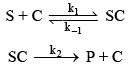Where S represents the reactant; C is catalyst and P is the product. The reactant or substrate-catalyst complex is represented by SC and is an intermediate.
The rate expression for product formation is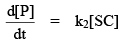…(1)

Because SC is an intermediate than apply S.S.A. on the formation of SC.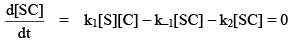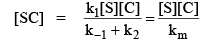…(2)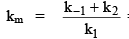= composite constant

then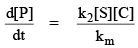…(3)

The relationship between initial concentration and the concentration of all species present after the reaction is:
[S]0 = [S] + [SC] + [P]
[C]0 = [C] + [SC]
then   [S] = [S]– [SC] – [P]               …(4)
&        [C] = [C]0 – [SC]                             …(5)
Substituting these values in equation (2), we get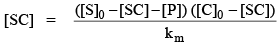Km[SC] = ([S]0 – [SC] – [P]) ([C]0 – [SC])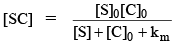then rate of the reaction becomes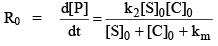Case I.

[C]0 <<  [S]0

i.e. much more substrate is present in comparison to catalyst. Then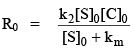if                           km < [S]0

Then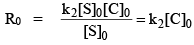i.e. zero order reaction with respect to substrate.

&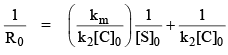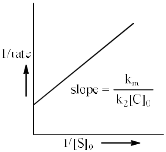when concentration of substrate [S]0 >> km then reaction rate

R0 = k2[C]0 = Rmax

i.e. the rate of reaction will reach a limiting value where the rate becomes zero order in substrate concentration.

Case II.

[C]0 >>  [S]0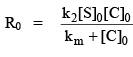i.e. the reaction rate is first order in [S]0, but can be first or zero order in [C]0 depending on the size of [C]relative to km.

Michaelis-Menten Enzyme Kinetics.

Enzyme are protein molecules that serve as catalysts in a chemical reaction.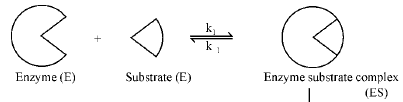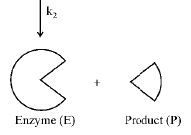The kinetic mechanism of enzyme catalyst can be described using the Michaelis-Menten mechanism.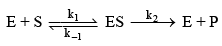The kinetic mechanism of enzyme catalyst can be described using the Michaelis-Menten mechanism.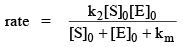But in this mechanism substrate concentration is greater than that of enzyme i.e.

[S]0 >> [E0]
then rate of formation of product in enzyme catalyst is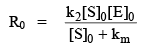…(1)

The co mposite constant km is referred to as the Michaelis constant in enzyme kinet ics and the equation is referred to as the Michaelis-Menten rate law.
When [S]0 >> km, the Michaelis constant can be neglected, resulting new expressio n for the rate.
R0 = k2[E]= Rmax

The reciprocal equation of equation (1) is the Lineweaver-Burk equation i.e.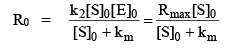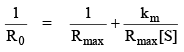…(2)

This equation is known as Lineweaver-Burk equation.
The plot of reciprocal of rate is known as Linewearver-Burk plot. k2 is known as turn over numberof the enzyme. “The turn over number is the maximum number of substrate molecules per uit time that can be converted into product.”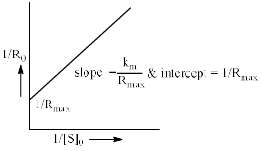This is Linewearver-Burk plot.
We know that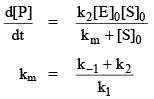Case I. ` [S]>> km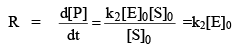i.e. rate is maximum due to all enzyme are present

R = Rmax = k2[E]0

This is zero order w.r.t. substrate.

Case II.      If                    [S]= km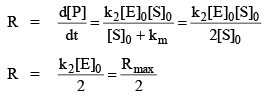Case III.       If                    [S] << km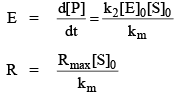This is first order w.r.t. substrate.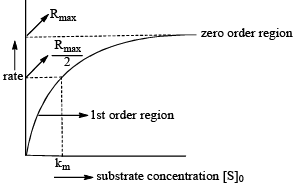This is graph between initial rate and concentration of substrate.

We know that,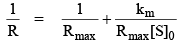Multiplying with R,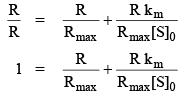Multiplying with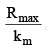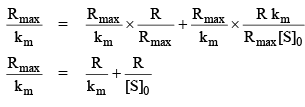or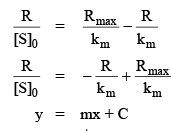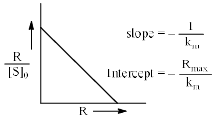Offer running on EduRev: Apply code STAYHOME200 to get INR 200 off on our premium plan EduRev Infinity!

## Physical Chemistry

77 videos|83 docs|32 tests

,

,

,

,

,

,

,

,

,

,

,

,

,

,

,

,

,

,

,

,

,

;